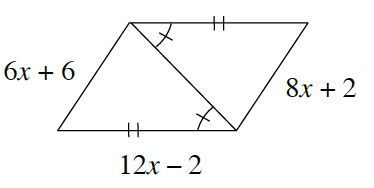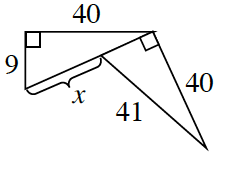### Home > GC > Chapter 7 > Lesson 7.2.4 > Problem7-74

7-74.

For each figure below, determine if the two smaller triangles in each figure are congruent. If so, explain why and solve for $x$. If not, explain why not.

Each set of triangles is congruent. Now show why or how.

1.Congruent because of $\text{SAS}≅$, $x=2$.

1.Congruent because of $\text{HL}≅$, $x=32$.